# Test: Binomial Theorem For Positive Index

## 15 Questions MCQ Test Mathematics (Maths) Class 11 | Test: Binomial Theorem For Positive Index

Description
This mock test of Test: Binomial Theorem For Positive Index for JEE helps you for every JEE entrance exam. This contains 15 Multiple Choice Questions for JEE Test: Binomial Theorem For Positive Index (mcq) to study with solutions a complete question bank. The solved questions answers in this Test: Binomial Theorem For Positive Index quiz give you a good mix of easy questions and tough questions. JEE students definitely take this Test: Binomial Theorem For Positive Index exercise for a better result in the exam. You can find other Test: Binomial Theorem For Positive Index extra questions, long questions & short questions for JEE on EduRev as well by searching above.
QUESTION: 1

### Find the value of r, if the coefficients of (2r + 4)th and (r – 2)th terms in the expansion of (1 + x)18 are equal.

Solution: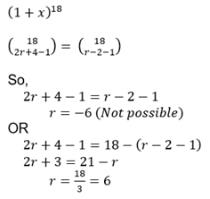QUESTION: 2

### The sixth term in the expansion of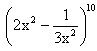is

Solution:

(2x2 - 1/3x2)10
T(6)  = T(5+1) = 10C5(2x2)5 (-1/3x2)5
= - 10!/(5!)(5!) * (2)5 * (1/(3)5
= - 10!/(5!)(5!)]32/243
= - 896/27

QUESTION: 3

### If the coefficients of 7th and 13th terms in the expansion of (1 + x)n are equal, then n is equal to

Solution:

Coefficent of 7th term is nC6 and 13th term is nC12
nC6 = nC12
⇒ nC(n-6) = nC12
n-6 = 12
n = 18

QUESTION: 4

The 6th term in the expansion of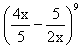is

Solution:

(4x/5−5/2x)9
Tr+1 in the expansion of (x+a)n is given by:
Tr+1=nCr x(n−r).ar
Hence 6th term will be given by:
T6=T5+1 = 9C5 (4x/5)4(−5/2x)5
=−(9.8.7.6.5)/(5.4.3.2.1).[44.x4]/54.[55/(25.x5)]
=−(9.8.7.6.5)/(5.4.3.2.1).[(44.x4)/54].[55/(25.x5)]
=−126(28).(5/25.x)
=−(630×23)/x
=−(5040)/x

QUESTION: 5

What is the coefficient of x5 in the expansion of (1-x)-6 ?

Solution:

(1-x)-6
=> (1-x)(-6/1)
It is in the form of (1-x)(-p/q), p =6, q=1
(1-x)(-p/q) = 1+p/1!(x/q)1 + p(p+q)/2!(x/q)2 + p(p+q)(p+2q)/3!(x/q)3 + p(p+q)(p+2q)(p+3q)/4!(x/q)4........
= 1+6/1!(x/1)1 + 6(7)/2!(x/1)2 + 6(7)(8)/3!(x/1)3 + 6(7)(8)(9)/4!(x/1)4 +.......................
So, coefficient of x5 is (6*7*8*9*10)/120
= 252

QUESTION: 6

In the expansion of the binomial expansion (a + b)n, which of the following is incorrect ?

Solution:

Explanation:- The coefficient of terms (x+a)^n equidistant from the beginning and the end are equal. These coefficients are known as the binomial coefficients and

nCr = nCn – r, r = 0,1,2,…,n.

QUESTION: 7

The middle term in the expansion of (x + y)10 is the

Solution:

Number of terms(n) = 10
Middle term = (n/2) + 1
= (10/2) + 1
⇒ 5 + 1
= 6th term

QUESTION: 8

In a binomial expansion with power 13

Solution: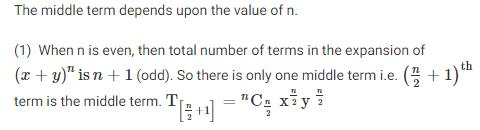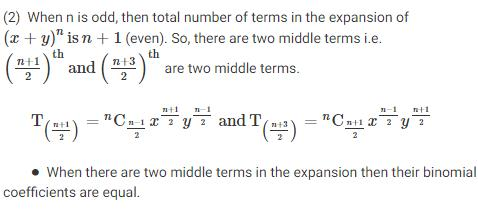QUESTION: 9

The number of terms in the expansion of (2x - 3y)8 is

Solution:

Since this binomial is to the power 8, there will be nine terms in the expansion.

QUESTION: 10

In the expansion of (a+b)n, nN the number of terms is:

Solution:

The total number of terms in the binomial expansion of (a + b)n is n + 1, i.e. one more than the exponent n.

QUESTION: 11

The middle term in the expansion of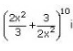Solution:

n = 10
Middle term = (n/2) + 1
= (10/2) + 1
= 6th term
T(6) = T(5+1) = 10C5[(2x2)/3]5 [(3/2x2)]5
= 10C5
= 252

QUESTION: 12

If in the expansion of (1+x)20, the coefficients of rth and (r+4)th terms are equal, then the value of r is equal to:

Solution:

Coefficients of the rth and (r+4)th terms in the given expansion are Cr−120  and 20Cr+3.
Here,Cr−120  = 20Cr+3⇒ r−1+r+3=20  [∵ if nCx=nCy  ⇒ x=y or x+y=n]
⇒r=2 or 2r=18
⇒r=9

QUESTION: 13

The number of terms in the expansion of (x – y + 2z)7 are:

Solution:
QUESTION: 14

The number of terms in the expansion of (a + b + c)n are:

Solution:

No. of terms is n+2C2

QUESTION: 15

The general term in the expansion of (a - b)n is

Solution:

If a and b are real numbers and n is a positive integer, then
(a - b)n = nC0 an + nC1 a(n – 1) b1 + nC2 a(n – 2) b2+ ...... + nCr a(n – r) br+ ... + nCnbn,
The general term or (r + 1)th term in the expansion is given by
Tr + 1 = (-1)Cr a(n–r) br